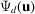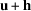International
Tables for
Crystallography
Volume B
Reciprocal space
Edited by U. Shmueli

International Tables for Crystallography (2006). Vol. B, ch. 4.3, p. 447   | 1 | 2 |

Section 4.3.5. Multislice calculations for diffraction and imaging

J. M. Cowleya and J. K. Gjønnesb

aArizona State University, Box 871504, Department of Physics and Astronomy, Tempe, AZ 85287-1504, USA, and  bInstitute of Physics, University of Oslo, PO Box 1048, N-0316 Oslo 3, Norway

4.3.5. Multislice calculations for diffraction and imaging

| top | pdf |

The description of dynamical diffraction in terms of the progression of a wave through successive thin slices of a crystal (Chapter 5.2) forms the basis for the multislice method for the calculation of electron-diffraction patterns and electron-microscope images [see Section 4.3.6.1in IT C (2004)]. This method can be applied directly to the calculations of diffuse scattering in electron diffraction due to thermal motion and positional disorder and for calculating the images of defects in crystals.

It is essentially an amplitude calculation based on the formulation of equation (4.3.4.1)[or (4.3.4.2)] for first-order diffuse scattering. The Bragg scattering in the first part of the crystal is calculated using a standard multislice method for the set of beams h. In the nth slice of the crystal, a diffuse-scattering amplitudeis convoluted with the incident set of Bragg beams. For each u, propagation of the set of beamsis then calculated through the remaining slices of the crystal. The intensities for the exit wave at the set of pointsare then calculated by adding either amplitudes or intensities. Amplitudes are added if there is correlation between the defects in successive slices. Intensities are added if there are no such correlations. The process is repeated for all u values to obtain a complete mapping of the diffuse scattering.

Calculations have been made in this way, for example, for short-range order in alloys (Fisher, 1969) and also for TDS on the assumption of both correlated and uncorrelated atomic motions (Doyle, 1969). The effects of the correlations were shown to be small.

This computing method is not practical for electron-microscope images in which individual defects are to be imaged. The perturbations of the exit wavefunction due to individual defects (vacancies, replaced atoms, displaced atoms) or small groups of defects may then be calculated with arbitrary accuracy by use of the periodic continuation' form of the multislice computer programs in which an artificial, large, superlattice unit cell is assumed [Section 4.3.6.1in IT C (2004)]. The corresponding images and microdiffraction patterns from the individual defects or clusters may then be calculated (Fields & Cowley, 1978). A more recent discussion of the image calculations, particularly in relation to thermal diffuse scattering, is given by Cowley (1988).

In order to calculate the diffuse-scattering distributions from disordered systems or from a crystal with atoms in thermal motion by the multislice method with periodic continuation, it would be necessary to calculate for a number of different defect configurations sufficiently large to provide an adequate representation of the statistics of the disordered system. However, it has been shown by Cowley & Fields (1979) that, if the single-diffuse-scattering approximation is made, the perturbations of the exit wave due to individual defects are characteristic of the defect type and of the slice number and may be added, so that a considerable simplification of the computing process is possible. Methods for calculating diffuse scattering in electron-diffraction patterns using the multislice approach are described by Tanaka & Cowley (1987) and Cowley (1989). Loane et al. (1991) introduced the concept of frozen phonons' for multislice calculations of thermal scattering.

References

International Tables for Crystallography (2004). Vol. C. Mathematical, physical and chemical tables, edited by E. Prince. Dordrecht: Kluwer Academic Publishers.Google Scholar
Cowley, J. M. (1988). Electron microscopy of crystals with time-dependent perturbations. Acta Cryst. A44, 847–853.Google Scholar
Cowley, J. M. (1989). Multislice methods for surface diffraction and inelastic scattering. In Computer simulation of electron microscope diffraction and images, edited by W. Krakow & M. O'Keefe, pp. 1–12. Worrendale, PA: The Minerals, Metals and Materials Society.Google Scholar
Cowley, J. M. & Fields, P. M. (1979). Dynamical theory for electron scattering from crystal defects and disorder. Acta Cryst. A35, 28–37.Google Scholar
Doyle, P. A. (1969). Dynamical calculation of thermal diffuse scattering. Acta Cryst. A25, 569–577.Google Scholar
Fields, P. M. & Cowley, J. M. (1978). Computed electron microscope images of atomic defects in f.c.c. metals. Acta Cryst. A34, 103–112.Google Scholar
Fisher, P. M. J. (1969). The development and application of an n-beam dynamic methodology in electron diffraction. PhD thesis, University of Melbourne. [See also Cowley (1981), ch. 17.]Google Scholar
Loane, R. F., Xu, P. R. & Silcox, J. (1991). Thermal vibrations in convergent-beam electron diffraction. Acta Cryst. A47, 267–278.Google Scholar
Tanaka, N. & Cowley, J. M. (1987). Electron microscope imaging of short range order in disordered alloys. Acta Cryst. A43, 337–346.Google Scholar